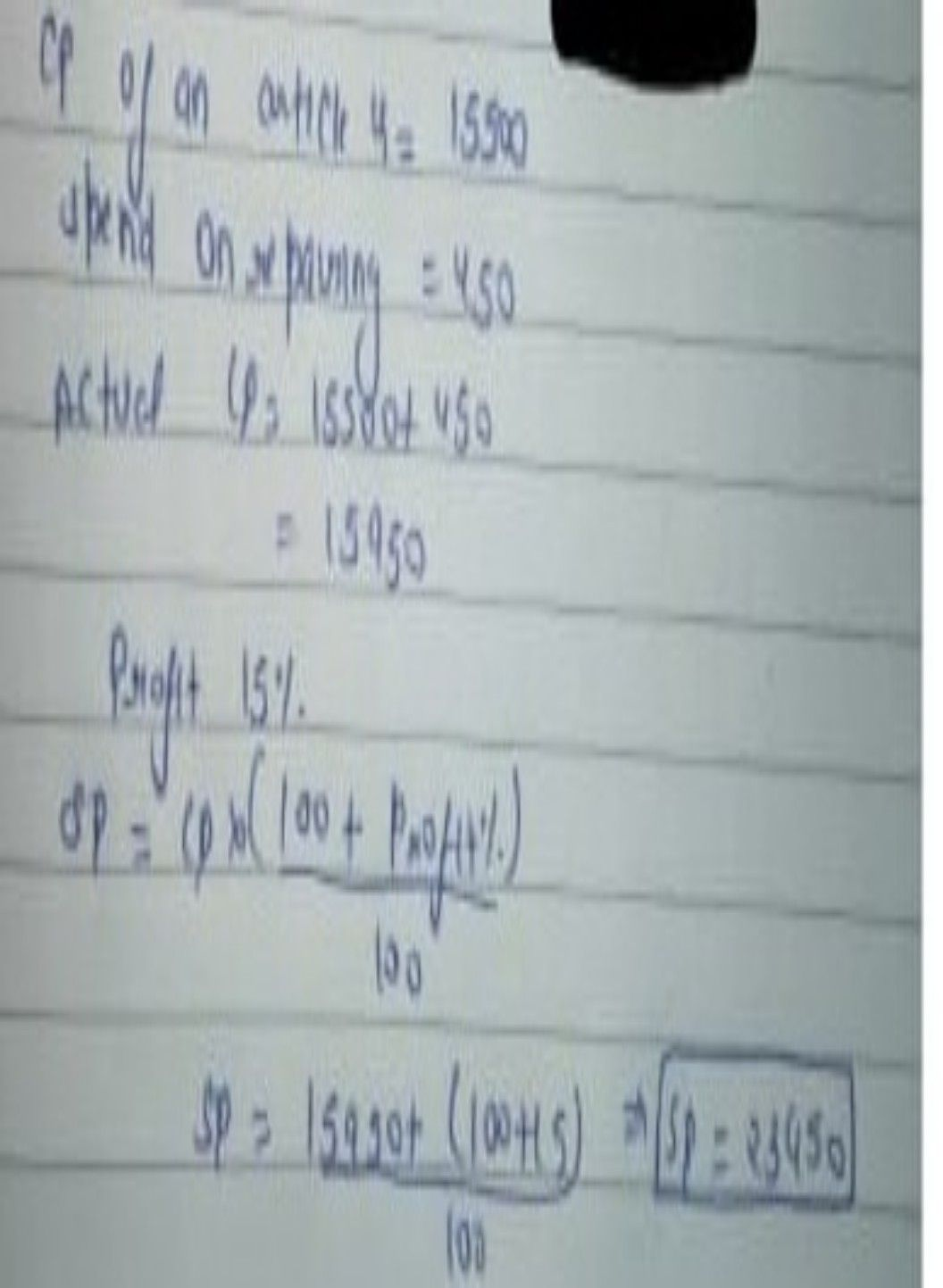Symbol
Problem$7$ $1$ $c0st-Dric$ $0^{fa}$ calculator becomes $6.4$ times $00$ itself and $sclling$ price $als0$ increases $b\right)$ $450%$ then new profit percentage $i6$ 76%.find $iniia|$ profit %?
Probability and Statistics
SolutionQanda teacher - lezlycrossplease do evaluate and please gift me coins